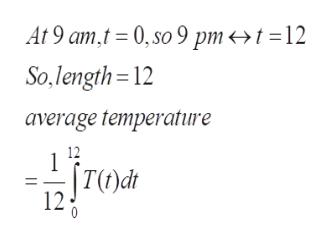In a certain city the temperature (in °F) t hours after 9 AM was modeled by the functionT(t) = 44 + 11 sin(pit/12)Find the average temperature Tave during the period from 9 AM to 9 PM. (Round your answer to the nearest whole number.)

Question

In a certain city the temperature (in °F) t hours after 9 AM was modeled by the function

T(t) = 44 + 11 sin(pit/12)

Find the average temperature Tave during the period from 9 AM to 9 PM. (Round your answer to the nearest whole number.)

Step 1

To calculate the average temperature using integration

Step 2

If we are given finitely many data points, we sum and take the average  to find the mean . In the continuous case, we take the integral and divide by the length to obtain the mean.

Step 3help_outlineImage TranscriptioncloseAt 9 amt 0,so 9 pm<>t =12 So,length=12 average temperature 12 1 |T)dt 12 fullscreen

Want to see the full answer?

See Solution

Want to see this answer and more?

Our solutions are written by experts, many with advanced degrees, and available 24/7

See Solution
Tagged in

Integration